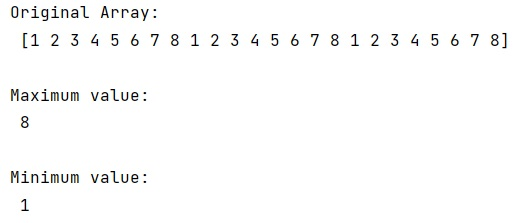# NumPy: function for simultaneous max() and min()

Learn, how to define a function for simultaneous max and min values in a numpy array?
Submitted by Pranit Sharma, on December 27, 2022

NumPy is an abbreviated form of Numerical Python. It is used for different types of scientific operations in python. Numpy is a vast library in python which is used for almost every kind of scientific or mathematical operation. It is itself an array which is a collection of various methods and functions for processing the arrays.

## Function for simultaneous max() and min()

For finding max and min values using NumPy, we have numpy.amax() and numpy.amin() methods. If we want to find both values, we need to call both methods which results in slow computation.

Hence, we will use another approach for this purpose. We will first store the first element of the array in a variable and then we will find the maximum and minimum element by comparing the first element with all the other elements.

Let us understand with the help of an example,

## Python code to demonstrate the function for simultaneous max() and min()

```# Import numpy
import numpy as np

# Creating a numpy array
arr = np.array([1,2,3,4,5,6,7,8,1,2,3,4,5,6,7,8,1,2,3,4,5,6,7,8])

# Display original array
print("Original Array:\n",arr,"\n")

# Storing first element
min = arr
max = arr

# Finding max and min value
for i in arr:
if i < min:
min = i
if i > max:
max = i

# Display the result
print("Maximum value:\n",max,"\n")
print("Minimum value:\n",min,"\n")
```

Output: